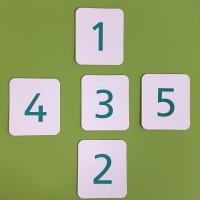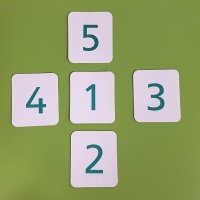#### You may also like### Counting Factors

Is there an efficient way to work out how many factors a large number has?### Summing Consecutive Numbers

15 = 7 + 8 and 10 = 1 + 2 + 3 + 4. Can you say which numbers can be expressed as the sum of two or more consecutive integers?### Helen's Conjecture

Helen made the conjecture that "every multiple of six has more factors than the two numbers either side of it". Is this conjecture true?

# Magic Crosses

##### Age 7 to 14 Challenge Level:
Here are pictures of two crosses:What do you notice about the two crosses?
What is the same?
What is different?

Both crosses use the digits 1, 2, 3, 4, and 5.
The left cross has a horizontal total of 12 and a vertical total of 6.
The right cross has a horizontal total of 8 and a vertical total of 8.

We call the cross on the right a magic cross, because its horizontal total is equal to its vertical total.

Can you find any more magic crosses using the digits 1, 2, 3, 4 and 5?
Can you find all the possibilities?
Can you convince yourself you have found them all?

You may wish to use the Magic Cross Tester to try out your ideas.

Here are some questions you might like to consider:

Are there any magic crosses that use the numbers 2, 3, 4, 5, 6?
Or 3, 4, 5, 6, 7?
Or 98, 99, 100, 101, 102?

How many magic crosses can you find with the number 50 in the middle?

What if the numbers went up in 2s instead?
Or 3s?
Or 10s, or 20s, or 100s?

Perhaps you have some questions of your own that you would like to explore too!